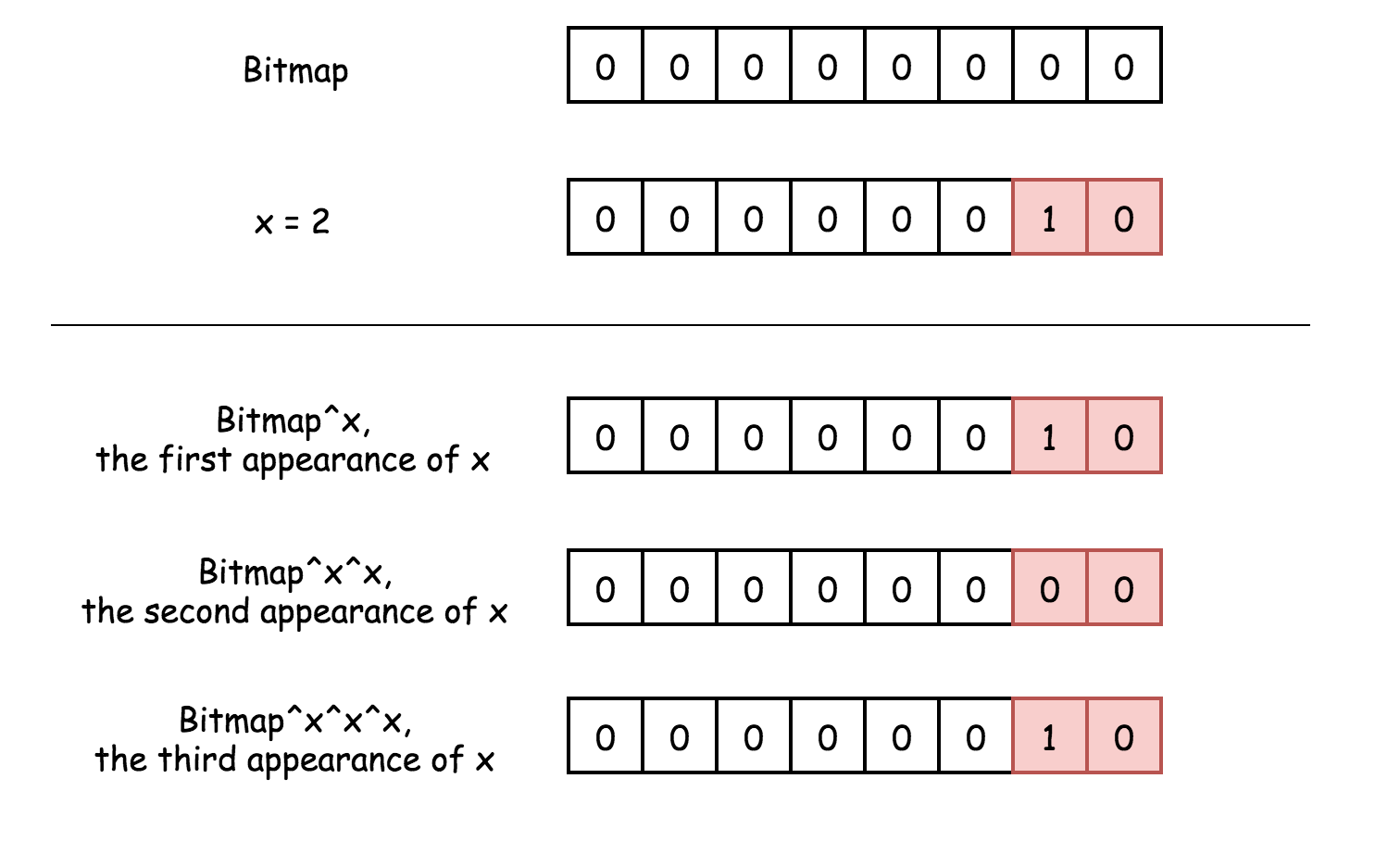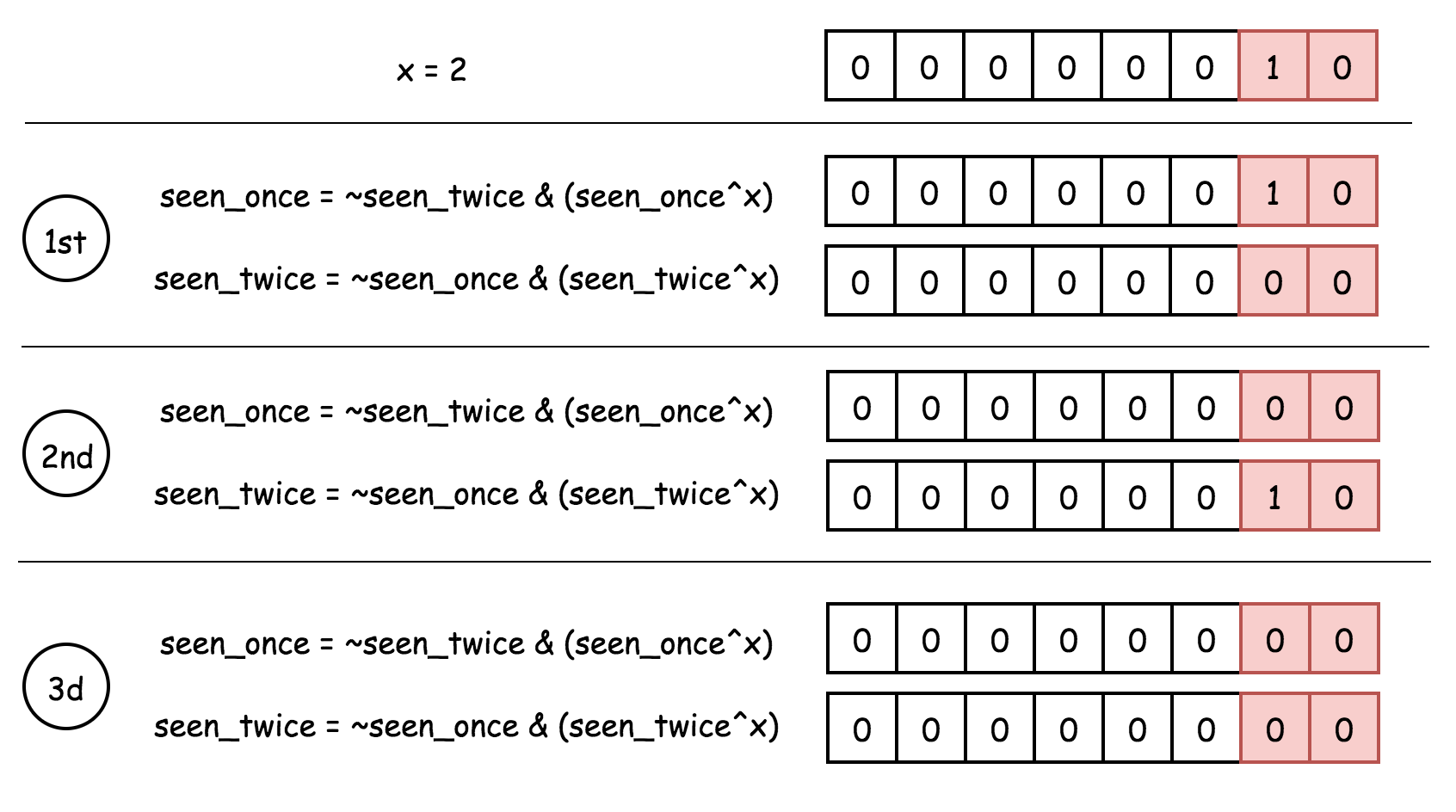# 题目

• 示例 1:
输入: [2,2,1]


• 示例 2:
输入: [4,1,2,1,2]



## 解题思路

public int singleNumber(int[] nums) {
if(nums.length == 1) {
return nums;
}

Map<Integer, Integer> map = new HashMap<>(nums.length);
for (int key : nums) {
if (map.containsKey(key)) {
map.remove(key);
} else {
map.put(key, 1);
}
}

//O(1)
for(Map.Entry<Integer, Integer> entry : map.entrySet()) {
return entry.getKey();
}
// 不应该到达
return -1;
}


Runtime: 8 ms, faster than 42.49% of Java online submissions for Single Number.
Memory Usage: 39.5 MB, less than 34.85% of Java online submissions for Single Number.


## 优化1-使用 HashSet

public int singleNumber(int[] nums) {
int result = 0;
// 能否進一步優化？
// hash 的優勢在於 O(1) 訪問。
// two pointer?
Set<Integer> set = new HashSet<>(nums.length);
for (int key : nums) {
if (set.contains(key)) {
result -= key;
} else {
result += key;
}
}
return result;
}


Runtime: 5 ms, faster than 54.71% of Java online submissions for Single Number.
Memory Usage: 39 MB, less than 83.31% of Java online submissions for Single Number.


## 优化2-位运算

a ^ a = 0
a ^ 0 = a


public int singleNumber(int[] nums) {
int result = 0;
for (int key : nums) {
result ^= key;
}
return result;
}


Runtime: 1 ms, faster than 95.67% of Java online submissions for Single Number.
Memory Usage: 39.3 MB, less than 48.69% of Java online submissions for Single Number.


### 更进一步优化

public int singleNumber(int[] nums) {
int result = nums;
for (int i = 1; i < nums.length; i++) {
result ^= nums[i];
}
return result;
}


# 只出现一次的数字 II

## 题目

• 示例 1:
输入: [2,2,3,2]


• 示例 2:
输入: [0,1,0,1,0,1,99]



## 解题思路-HashMap

public int singleNumber(int[] nums) {
// 參數防禦
if(nums == null || nums.length == 0) {
throw new IllegalArgumentException();
}
if(nums.length == 1) {
return nums;
}

Map<Integer, Integer> map = new HashMap<>(nums.length);
for(int i : nums) {
Integer count = map.get(i);
if(count == null) {
count = 0;
}
map.put(i, count+1);
}
// 遍歷
for(Map.Entry<Integer, Integer> entry : map.entrySet()) {
if(1 == entry.getValue()) {
return entry.getKey();
}
}

throw new IllegalArgumentException();
}


## 位运算

### 异或 XOR

• 0 与任何数 XOR 结果为该数。

• 两个相同的数 XOR 结果为 0。### AND NOT### java 实现

public int singleNumber(int[] nums) {
int seenOnce = 0, seenTwice = 0;

for (int num : nums) {
// first appearence:
// don't add to seen_twice because of presence in seen_once
// second appearance:
// remove num from seen_once
// third appearance:
// don't add to seen_once because of presence in seen_twice
// remove num from seen_twice
seenOnce = ~seenTwice & (seenOnce ^ num);
seenTwice = ~seenOnce & (seenTwice ^ num);
}
return seenOnce;
}


第一次出现时，once和twice均为0，once^num相当于把num添加到once，表示num出现了一次，~once表示不把num添加到twice；



Runtime: 0 ms, faster than 100.00% of Java online submissions for Single Number II.
Memory Usage: 38.8 MB, less than 41.37% of Java online submissions for Single Number II.


# 只出现一次的数字 III

## 題目

• 示例 1：

• 示例 2：

• 示例 3：

2 <= nums.length <= 3 * 104

-2^31 <= nums[i] <= 2^31 - 1

## 解題思路

public int[] singleNumber(int[] nums) {
Map<Integer, Integer> map = new HashMap<>(nums.length);
for(int num : nums) {
map.put(num, map.getOrDefault(num, 0)+1);
}
// 獲取結果
int[] results = new int;
int size = 0;
for(Map.Entry<Integer, Integer> entry : map.entrySet()) {
if(entry.getValue() == 1) {
results[size++] = entry.getKey();
}
}
return results;
}




Runtime: 4 ms, faster than 28.51% of Java online submissions for Single Number III. Memory Usage: 40.3 MB, less than 24.68% of Java online submissions for Single Number III.



## 优化思路-位运算

### 核心思路

（1）相同的数，依然在相同组。

（2）唯一出现一次的数，被分到 2 个组。

### java 实现

（1）全部异或一遍，找到两个出现一次的 XOR 结果。

（2）找到 XOR 非零位

（3）以 XOR 的非零位对原始数组进行划分为 2 个部分，分别进行 XOR

java
public int[] singleNumber(int[] nums) {
int xor = 0;
for(int num : nums) {
xor ^= num;
}

// 获取非0位
int bit = 1;
while ((bit & xor) == 0) {
bit <<= 1;
}

// 重新划分数组
int a = 0;
int b = 0;
for(int num : nums) {
if((num & bit) == 0) {
a ^= num;
} else {
b ^= num;
}
}
return new int[]{a, b};
}


Runtime: 1 ms, faster than 96.60% of Java online submissions for Single Number III.
Memory Usage: 39 MB, less than 73.26% of Java online submissions for Single Number III.


## 优化2-尽头

a XOR b = xor


b = xor ^ a


### java 实现

public int[] singleNumber(int[] nums) {
int xor = 0;
for(int num : nums) {
xor ^= num;
}

// 获取非0位
int bit = 1;
while ((bit & xor) == 0) {
bit <<= 1;
}

// 重新划分数组
int a = 0;
for(int num : nums) {
if((num & bit) == 0) {
a ^= num;
}
}
// a ^ xor 就是另一个数
return new int[]{a, a ^ xor};
}


Runtime: 0 ms, faster than 100.00% of Java online submissions for Single Number III.
Memory Usage: 39 MB, less than 82.62% of Java online submissions for Single Number III.


# 参考资料

https://leetcode-cn.com/problems/single-number